Consider allowing JavaScript. Otherwise, you have to be proficient in readingsince formulas will not be rendered. Furthermore, the table of contents in the left column for navigation will not be available and code-folding not supported. Sorry for the inconvenience.

Examples in this article were generated with4.2.0 by the package PowerTOST.1 More examples are given in the respective vignette.2 See also the README on GitHub for an overview and the online manual3 for details.

• The right-hand badges give the respective section’s ‘level’.

1. Basics about sample size methodology – requiring no or only limited statistical expertise.

1. These sections are the most important ones. They are – hopefully – easily comprehensible even for novices.

1. A somewhat higher knowledge of statistics and/or R is required. May be skipped or reserved for a later reading.
• Click to show / hide R code.

# Introduction

What is the purpose of a Power Analysis?

Since it is extremely unlikely that we will observe exactly our assumed values in a study, in a – prospective – power analysis we explore the impact of potential deviations from our assumptions on power.

Prospective (a priori, ante hoc) power must not be confused with the – futile4 5 6 7 8 – retrospective (a posteriori, post hoc) power. The latter is elaborated in another article.

## Preliminaries

A basic knowledge of R does not hurt. To run the scripts at least version 1.2-1 (2014-09-30) of PowerTOST is required and for the GCC’s approach at least version 1.5-3 (2021-01-18). Any version of R would likely do, though the current release of PowerTOST was only tested with version 4.1.3 (2022-03-10) and later. All scripts were run on a Xeon E3-1245v3 @ 3.40GHz (1/4 cores) 16GB RAM with R 4.2.0 on Win­dows 7 build 7601, Service Pack 1, Universal C Runtime 10.0.10240.16390.

Note that in all functions of PowerTOST the arguments (say, the assumed T/R-ratio theta0, the BE-limits (theta1, theta2), the assumed coefficient of variation CV, etc.) have to be given as ratios and not in percent.

Sample sizes are given for equally sized groups (parallel design) and for balanced sequences (crossovers, replicate designs). Furthermore, the estimated sample size is the total number of subjects, which is always an even number (parallel design) or a multiple of the number of sequence (crossovers, replicate designs) – not subjects per group or sequence like in some other software packages.

## Terminology

In order to get prospective power (and hence, a sample size), we need five values:

1. The level of the test $$\small{\alpha}$$ (in BE commonly 0.05),
2. the BE-margins (commonly 0.80 – 1.25),
3. the desired (or target) power $$\small{\pi}$$,
4. the variance (commonly expressed as a coefficient of variation), and
5. the deviation of the test from the reference treatment.

1 – 2 are fixed by the agency,
3 is set by the sponsor (commonly to 0.80 – 0.90), and
4 – 5 are just (uncertain!) assumptions.

Realization: Observations (in a sample) of a random variable (of the population).

Since it is extremely unlikely that all assumptions will be exactly realized in a particular study, it is worthwhile to prospectively explore the impact of potential deviations from assumptions on power.

We don’t have to be concerned about values which are ‘better’ (i.e., a lower CV, and/or a T/R-ratio closer to unity, and/or a lower than anticipated dropout-rate) because we will gain power. On the other hand, any change towards the ‘worse’ will negatively impact power.

# Sample size → Power

The sample size cannot be directly estimated, only power calculated for an already given sample size.

What we can do: Estimate the sample size based on the conditions given above and vary our assumptions (4 – 5) accordingly. Furthermore, we can decrease the sample size to explore the impact of dropouts on power.

# Examples

Let’s start with PowerTOST.

library(PowerTOST) # attach it to run the examples

Although we could do that ‘by hand’, there a three functions supporting power analyses: pa.ABE() for conventional Average Bioequivalence (ABE), pa.scABE() for Scaled Average Bioequivalence (in its variants ABEL and RSABE), and pa.NTID() for RSABE of NTIDs.

Note that the functions support only homoscedasticity ($$\small{CV_\textrm{wT}\equiv CV_\textrm{wR}}$$).

The defaults common to all functions are:

Argument Default Meaning
alpha 0.05 Nominal level of the test $$\small{\alpha}$$, probability of the Type I Error (patient’s risk)
targetpower 0.80 Target (desired) power $$\small{\pi = 1-\beta}$$, where $$\small{\beta}$$ is the probability of the Type II Error (producer’s risk)
minpower 0.70 Minimum acceptable power
theta1 0.80 Lower BE-limit in ABE and lower PE-constraint in SABE
theta2 1.25 Upper BE-limit in ABE and upper PE-constraint in SABE

The default of the assumed T/R-ratio theta0 is 0.95 in pa.ABE(), 0.90 in pa.scABE(), and 0.975 in pa.NTIDFDA(). In pa.ABE() any design can be specified (defaults to "2x2").
In pa.scABE() the default design is "2x3x3" and regulator = "EMA".
In pa.NTIDFDA() only a 2-sequence 4-period full replicate design is implemented in accordance with the FDA’s guidance.9 10

Let’s explore in the following examples given in other articles.

## Parallel Design

We assume a CV of 0.40, a T/R-ratio of 0.95, target a power of 0.80 (see also the example in the corresponding article about the parallel design), and keep the default minimum power 0.70.

CV <- 0.40
x  <- pa.ABE(CV = CV, design = "parallel") # assign to an object
dev.new(width = 6.2, height = 6.7)
plot(x, pct = FALSE, ratiolabel = "theta0")
par(op)
print(x, plot.it = FALSE)
# Sample size plan ABE
#    Design alpha  CV theta0 theta1 theta2 Sample size
#  parallel  0.05 0.4   0.95    0.8   1.25         130
#  Achieved power
#        0.803512
#
# Power analysis
# CV, theta0 and number of subjects leading to min. acceptable power of ~0.7:
#  CV= 0.4552, theta0= 0.9276
#  n = 103 (power= 0.701)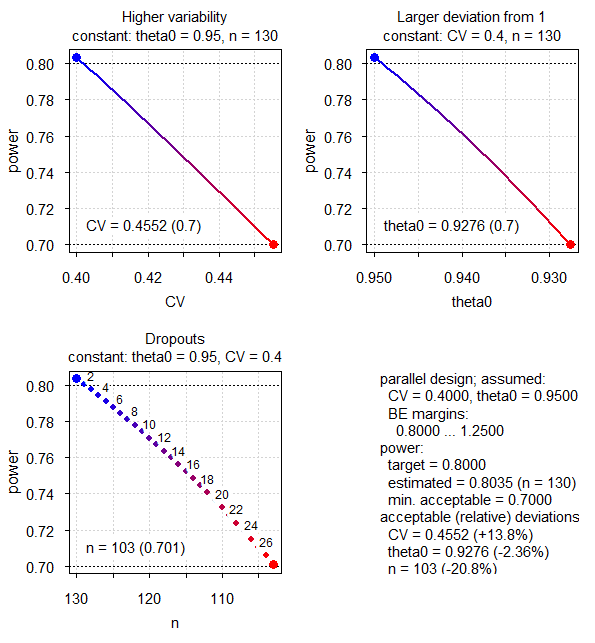Fig.1 Parallel design (CV 0.40, T/R ratio 0.95).

Such patterns are typical for ABE. Note that the x-axes of the second and third panels are reversed (decreasing).

The most sensitive parameter is the T/R-ratio $$\small{\theta_0}$$, which can decrease from the assumed 0.95 to 0.9276 (maximum relative deviation –2.36%) until we reach our minimum acceptable power of 0.70. The CV is much less sensitive, which can increase from the assumed 0.40 to 0.4552 (relative +13.8%). The least sensitive is the sample size itself, which can decrease from the planned 130 subjects to 103 (relative –20.8%). Hence, dropouts are in general of the least concern.

## Crossover Design

### Conventional ABE

We assume a CV of 0.25, a T/R-ratio of 0.95, target a power of 0.80 (see also the example in the corresponding article about the 2×2×2 design), and keep the default minimum power 0.70.

CV <- 0.25
x  <- pa.ABE(CV = CV)
dev.new(width = 6.2, height = 6.7)
plot(x, pct = FALSE, ratiolabel = "theta0")
par(op)Fig.2 2×2×2 design (CV 0.25, T/R ratio 0.95).

The order of parameters influencing power is as common in ABE: $$\small{\theta_0}$$ $$\small{\gg}$$ CV $$\small{>}$$ n.

### ABE for NTIDs

We assume a CV of 0.125, a T/R-ratio of 0.975, target a power of 0.80 (see also the example in the corresponding article about the 2×2×2 design), and keep the default minimum power 0.70.

CV     <- 0.125
theta0 <- 0.975
theta1 <- 0.90
x  <- pa.ABE(CV = CV, theta0 = theta0, theta1 = theta1)
dev.new(width = 6.2, height = 6.7)
plot(x, pct = FALSE, ratiolabel = "theta0")
par(op)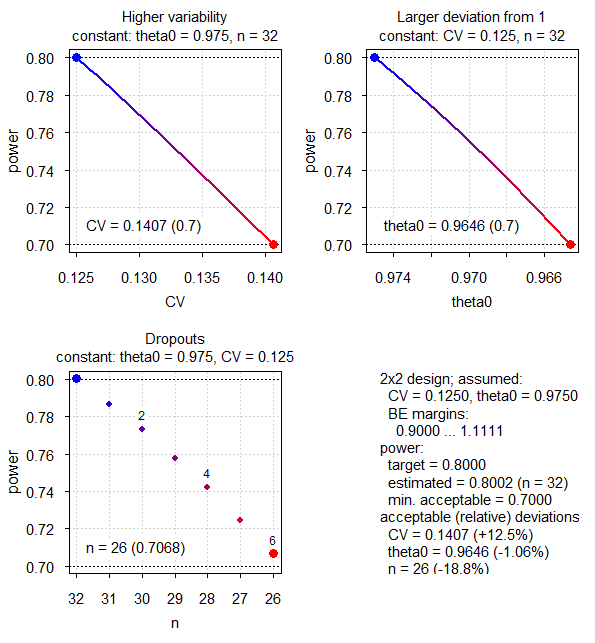Fig.3 2×2×2 design (CV 0.125, T/R ratio 0.975, NTID).

## HVDs / HVDPs

### ABEL

#### EMA and most others

We assume a CV of 0.45, a T/R-ratio of 0.90, target a power of 0.80 (see also the example in the corresponding article), keep the default minimum power 0.70, and want to perform the study in a 2-sequence 4-period full replicate study (TRTR|RTRT or TRRT|RTTR or TTRR|RRTT).

CV     <- 0.45
design <- "2x2x4"
x      <- pa.scABE(CV = CV, design = design)
dev.new(width = 6.2, height = 6.7)
plot(x, pct = FALSE, ratiolabel = "theta0")
par(op)Fig.3 2×2×4 replicate design (CV 0.45, T/R ratio 0.90, ABEL for the EMA).

Here the pattern is different to ABE. The idea of reference-scaling is to maintain power irrespective of the CV. Therefore, the CV is the least sensitive parameter – it can increase from the assumed 0.45 to 0.6629 (relative +47.3%). If the CV decreases, power decreases as well because we can expand limits less.

Let’s dive deeper. x is an S3 object.11 Contained in its list are the data.frames paCV, paGMR, and paN. With the function power.scABEL() we can assess the components of power at specific values of the CV.

n       <- x$paN$N
max.pwr <- which(x$paCV$pwr == max(x$paCV$pwr))
CVs     <- c(head(x$paCV$CV, 1),
x$paCV$CV[x$paCV$pwr == min(x$paCV$pwr[1:max.pwr])],
CV, x$paCV$CV[max.pwr], tail(x$paCV$CV, 1))
res     <- data.frame(CV = CVs, V1 = NA, V2 = NA, V3 = NA, V4 = NA)
for (i in 1:nrow(res)) {
res[i, 2:5] <- suppressMessages(
power.scABEL(CV = CVs[i], design = design,
n = n, details = TRUE))
}
names(res)[2:5] <- c("p(BE)", "p(BE-ABEL)",
"p(BE-PE)", "p(BE-ABE)")
print(signif(res, 4), row.names = FALSE)
#      CV  p(BE) p(BE-ABEL) p(BE-PE) p(BE-ABE)
#  0.3000 0.7383     0.7383   0.9832    0.6786
#  0.3167 0.7351     0.7351   0.9784    0.6403
#  0.4500 0.8112     0.8116   0.9266    0.4107
#  0.4766 0.8164     0.8165   0.9161    0.3764
#  0.6629 0.7000     0.7000   0.8467    0.1546

p(BE) is the overall power, p(BE-ABEL) is the power of ‘pure’ ABEL (without the PE-restriction), and p(BE-PE) is the power of the criterion ‘point estimate within acceptance range’ alone. p(BE-ABE) is the power of the conventional ABE test for comparative purposes.

We see that close to the upper cap of scaling (at CV 50%) power starts to decrease because we cannot expand the limits any more (maximum expansion 69.84 – 143-19%). Furthermore, the PE-restriction becomes increasingly important.

As above but according to the rules of Health Canada (upper cap of scaling ~57.4% instead of 50%).12

CV     <- 0.45
design <- "2x2x4"
x      <- pa.scABE(CV = CV, design = design,
regulator = "HC")
dev.new(width = 6.2, height = 6.7)
plot(x, pct = FALSE, ratiolabel = "theta0")
par(op)Fig.4 2×2×4 replicate design (CV 0.45, T/R ratio 0.90, ABEL for Health Canada).

Similar to the EMA but more relaxed due to the higher upper cap.

#### GCC

That’s a special case because for any CV > 30% the limits are directly widened to 75.00 – 133.33% and there is no upper cap of scaling.13

CV     <- 0.45 # at least v1.5.3 of PowerTOST required
design <- "2x2x4"
x      <- pa.scABE(CV = CV, design = design,
regulator = "GCC")
dev.new(width = 6.2, height = 6.7)
plot(x, pct = FALSE, ratiolabel = "theta0")
par(op)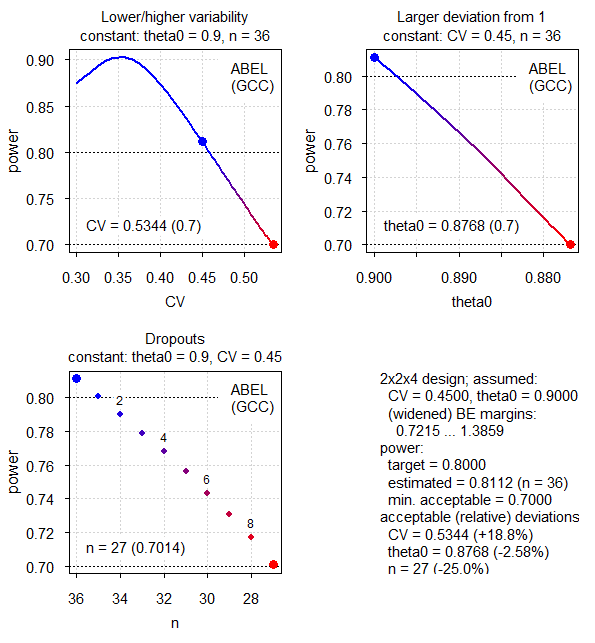Fig.5 2×2×4 replicate design (CV 0.45, T/R ratio 0.90, ABEL for the GCC).

### RSABE

As above but for the U.S. FDA and China’s CDE (see also the example in the corresponding article).

CV     <- 0.45
design <- "2x2x4"
x      <- pa.scABE(CV = CV, design = design,
regulator = "FDA")
dev.new(width = 6.2, height = 6.7)
plot(x, pct = FALSE, ratiolabel = "theta0")
par(op)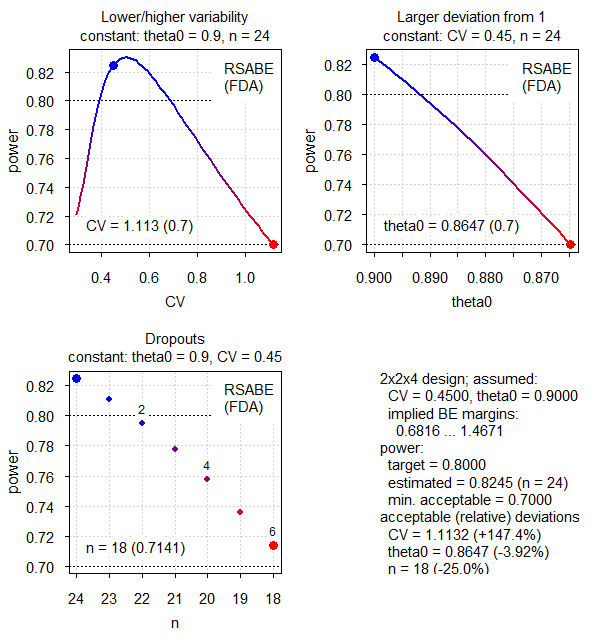Fig.6 2×2×4 replicate design (CV 0.45, T/R ratio 0.90, RSABE).

Due to unlimited scaling the CV is even less important than in the other methods.

## NTIDs

We assume a CV of 0.125, a T/R-ratio of 0.975 (the function’s default), target a power of 0.80, and want to perform the study in a 2-sequence 4-period full replicate study (TRTR|RTRT or TRRT|RTTR or TTRR|RRTT). Note that such a design is mandatory for the FDA and China’s CDE. See also the example in the corresponding article.

CV     <- 0.125
design <- "2x2x4"
x      <- pa.NTID(CV = CV, design = design)
dev.new(width = 6.2, height = 6.9)
plot(x, pct = FALSE, ratiolabel = "theta0")
par(op)Fig.7 2×2×4 replicate design (CV 0.125, T/R ratio 0.975, RSABE for NTIDs).

Here the CV shows a different behavior to both RSABE for HVDs / HVDPs and ABE (see above). Maximum power is seen at ~20.4% and we observe two minima.

# Cave!

A power analysis is not a substitute for the ‘Sensitivity Analysis’ recommended by the ICH14 because in a real study a combination of all effects occurs simultaneously.
It is up to you to decide on reasonable combinations and analyze their respective power.

How to explore deviations simultaneously is elaborated in another article.Helmut Schütz 2022
R and PowerTOST GPL 3.0, pandoc GPL 2.0.
1st version April 11, 2021. Rendered June 21, 2022 11:24 CEST by rmarkdown via pandoc in 0.58 seconds.

Footnotes and References

1. Labes D, Schütz H, Lang B. PowerTOST: Power and Sample Size for (Bio)Equivalence Studies. Package version 1.5.4. 2022-02-21. CRAN.↩︎

2. Schütz H. Power Analysis. 2021-02-21. CRAN.↩︎

3. Labes D, Schütz H, Lang B. Package ‘PowerTOST’. February 21, 2022. CRAN.↩︎

4. Lenth RV. Two Sample-Size Practices that I Don’t Recommend. October 24, 2000. Online.↩︎

5. Hoenig JM, Heisey DM. The Abuse of Power: The Pervasive Fallacy of Power Calculations for Data Analysis. Am Stat. 2010; 55(1): 19–24. doi:10.1198/000313001300339897.Open Access.↩︎

6. Lenth RL. Some Practical Guidelines for Effective Sample Size Determination. Am Stat. 2010; 55(3): 187–93. doi:10.1198/000313001317098149.↩︎

7. Senn S. Power is indeed irrelevant in interpreting completed studies. BMJ. 2002. 325(7375); 1304. PMID 12458264.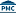Free Full Text.↩︎

8. WHO. Frequent deficiencies in BE study protocols. Geneva. November 2020. Online.↩︎

9. FDA, OGD. Draft Guidance on Warfarin Sodium. Recommended Dec 2012. Download.↩︎

10. FDA, CDER. Draft Guidance for Industry. Bioequivalence Studies with Pharmacokinetic Endpoints for Drugs Sub­mitted Under an ANDA. Rockville. August 2021. Download.↩︎

11. Wickham H. Advanced R. The S3 object system. 2019-08-08.↩︎

12. Health Canada. Guidance Document – Comparative Bioavailability Standards: Formulations Used for Systemic Effects. Ottawa. 2018/06/08. ISBN: 978‐0‐660‐25514‐9.↩︎

13. Executive Board of the Health Ministers’ Council for GCC States. The GCC Guidelines for Bioequivalence. May 2021. Online.↩︎

14. International Conference on Harmonisation of Technical Requirements for Registration of Phar­ma­ceu­ti­cals for Human Use. ICH Harmonised Tripartite Guideline. Statistical Principles for Clinical Trials. 5 February 1998. Online.↩︎